Matter

Humans have been observing and tracking the movements of celestial bodies for thousands of years, but have been spacefaring for just sixty. The possibility of space travel is a recent development in human history. Mostly, humans have been earthbound, with eyes skyward.The night sky with its vast patterns captures our attention. For multiple ancient civilizations, mythical characters contained in constellations acted out timeless adventures—the original nighttime TV lineup. The repeating celestial patterns eventually gave birth to early science. The purpose of this course is to appreciate these developments and open the door to a modern view of humans' place in the universe.

All astronomy begins with observations on Earth. The stuff that stars are made from is the same stuff that makes up our home planet. We are literally made of stardust. In this chapter, we will remind ourselves of some principles that are widely used in astronomy and astrophysics.

Matter

Earth harbors a somewhat rare environment as far as we can tell. We look around and see objects that are solid (like rocks and wood) and some liquids (mostly water), and we constantly breathe gaseous air. However, most places in our solar system are either too hot or too cold to observe solids, liquids, and gases simultaneously.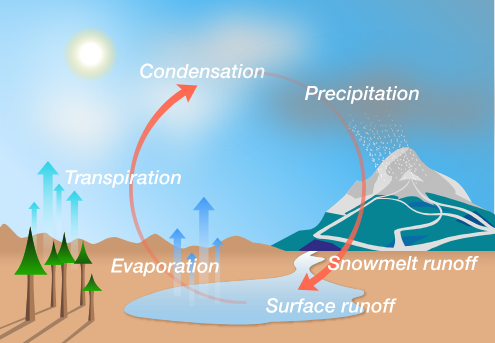All matter is characterized by its density. Average density $\rho$ is the amount of mass contained in a particular volume: $\rho = \frac{\text{Total mass}}{\text{Volume}}.$

Which state of matter usually has the lowest density?

Matter

Density varies widely depending on where you are in our solar system. On rocky bodies like Earth, density is relatively high. There are four rocky planets and thousands of rocky or icy minor planets orbiting our Sun. All solid, orbiting bodies share a similar density scale.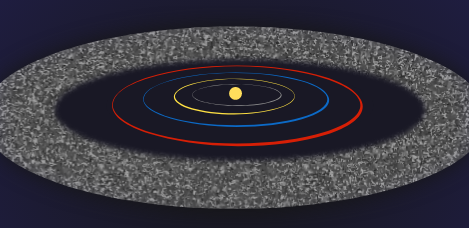What is a typical density $($in $\si[per-mode=symbol]{\kilo\gram\per\meter\cubed})$ of a rocky body in our solar system? Use Earth as a typical rocky body.


Details & Assumptions:

• The mass of the Earth is $M_\text{Earth}=\SI{5.97e24}{\kilo\gram}.$
• Assume the Earth is a sphere with radius $R_\text{Earth}=\SI{6.37e6}{\meter}.$
• A spherical Earth has volume $V=\frac{4}{3}\pi R_\text{Earth}^3.$

Matter

Temperature plays a large role in determining matter's phase, and thereby its density. Later in this course, we will develop a model for predicting a planet's temperature depending on the power of its parent star.

Temperature correlates to the average energy of molecules within a substance. It is often measured in degrees Celsius or Fahrenheit, but astronomers and physicists use Kelvin $(\si{\kelvin})$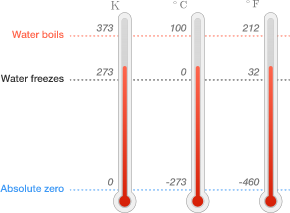Based on the comparison above, what do you think the average surface temperature $($in $\si{\kelvin})$ is on Earth?


Details and Assumptions:

• To convert degrees Celsius to $\si{\kelvin},$ you add $273.$
• The average temperature on Earth is taken over data from all possible locations, sampled over the whole year.

Matter

A material's density changes with its temperature—e.g., a piece of metal expands in direct sunlight, or a helium balloon contracts in the freezer. An equation of state relates the temperature of a material to other measurable variables like density.

Low-density gases have particularly simple equations of state because individual molecules have little influence on others. If we imagine a box of gas, the molecules bounce off the walls creating an outward gas pressure $P,$ but do not apply forces on each other. This simplified system is called an ideal gas.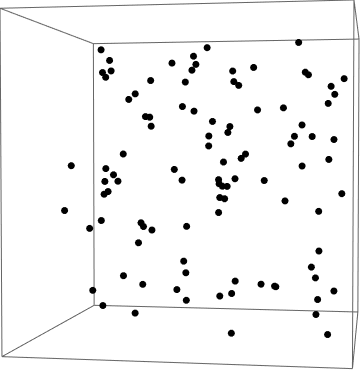In astronomy, we leverage this class of gas model wherever possible, so to close out this quiz, we will introduce its equation of state.

Matter

The ideal gas law relates the gas pressure $P$ to the density $\rho$ and temperature $T$: $P = \frac{\rho k_B T}{m},$ where $m$ is the mass of one gas molecule. Boltzmann's constant $k_B=\SI[per-mode=symbol]{1.381e-23}{\joule\per\kelvin}$ calibrates the Kelvin temperature scale to energy in Joules.

Use the ideal gas law to estimate the density of diatomic nitrogen $($in $\si[per-mode=symbol]{\kilo\gram\per\meter\cubed})$ at Earth's surface.


Details & Assumptions:

• The measured pressure at sea level on Earth's surface is $\SI{1.01e5}{\pascal}.$
• Take the temperature of the gas to be Earth's average temperature: $\SI{288}{\kelvin}.$
• Assume that Earth's atmosphere consists entirely of diatomic nitrogen, $\ce{N2}.$ The mass of a diatomic nitrogen $(\ce{N2})$ molecule is $\SI{4.65e-26}{kg}.$

Matter

The next quiz will introduce gravity, the universal force responsible for pulling matter together. Without gravity, the density of the universe would be essentially uniform (and very small).

To get a sense of how empty most of space is, compare our solar system's average density to Earth's atmosphere. The total mass of the solar system is $\SI{1.99e30}{\kilo\gram}.$ A conservative estimate of the radius of the solar system is $\SI{7.5e9}{\kilo\meter}.$ This is about $50$ times the distance from Earth to the Sun.

About how many times more dense is Earth's atmosphere than the solar system?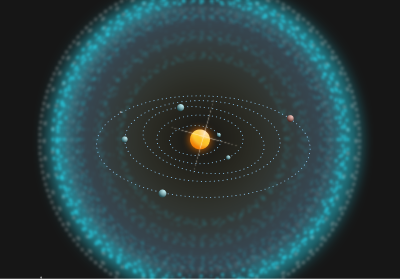Details and Assumptions:

• You can only compare two measurements if they have the same units. You just found that the Earth's atmosphere has a density slightly above $\SI[per-mode=symbol]{1}{\kilo\gram\per\meter\cubed}.$
• The Sun's influence can be felt in all directions, so assume the solar system is a sphere with volume $\frac43 \pi R^3$.

×